# Monitoring Multiple Processes on a Single Control Chart

#### Multiple Process Streams

In many industries, one is faced with the need to control multiple independent process streams producing the same desired output. In this example, we are monitoring a single filling machine with eight independent filling heads. (These heads are independent because there is a separate pump for each head.)

The 20 samples of data in Table 1 represent fill volume at each head. One sample is taken every 15 minutes.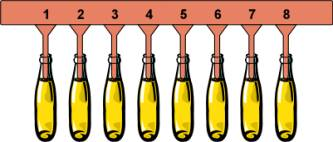Figure 1: Bottle filling machine with eight fill heads. Each head is controlled by a separate pump.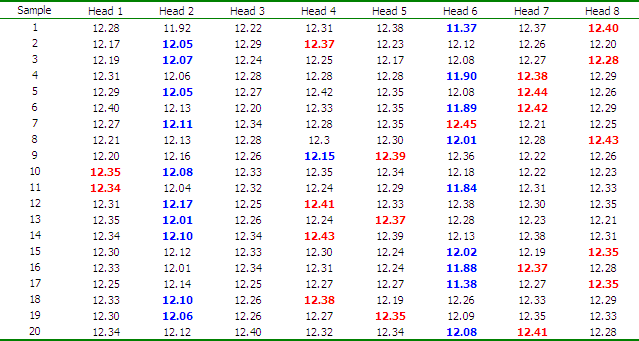Table 1: Data representing 20 samples. A sample of eight containers (one from each head) is taken every 15 minutes. Values in red are the MAX sample values and blue are the MIN values.

### Interpreting the Group Chart

For each sample, the MAX and MIN values are plotted on an individuals (IX) chart (Figure 2, top chart). The moving range values are calculated for each fill head between each sample. The largest and smallest moving ranges are then plotted as the MAX and MIN moving range values on the Moving R chart (Figure 2, bottom chart). For example, in sample 2, fill head 4 had the smallest moving range |12.31- 12.37| = 0.06 and fill head 6 had the largest |11.37- 12.12| = 0.75.

The centerlines represent the average from all the data, not just the plotted MAX and MIN values. The control limits, short term sigma and Cp/Cpk are based on the pooled standard deviations among all eight fill heads.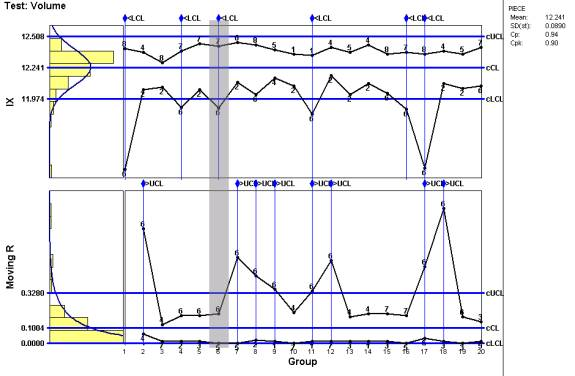Figure 2: Group IX-MR chart showing the MIN and MAX fill volume values (IX chart) and the MIN and MAX moving ranges from each sample (Moving R chart). Both charts contain several out of control plot points.

When interpreting a group chart, you look for runs in the MAX and MIN positions. On the IX chart, notice that the MIN plot points are predominately heads 2 and 6. This means that those heads are consistently filling less volume than the other heads. Also, the majority of the MAX moving range plot points are from head 6. This means that the variability from that head is consistently greater than the other heads.

### Viewing Multiple Process Streams on a Box Plot

Group charts are great for looking at the behavior of multiple process streams over time. Another tool used to compare multiple distributions is the Box Plot. Like a histogram, the Box Plot is illustrating a data's distribution (Figure 3). A histogram is the front view, a Box Plot is the top view.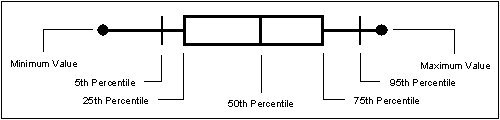Figure 3: Box Plot Interpretation

Notice that the Box Plot clearly shows that heads 2 and 6 are filling less volume than the others (Figure 4). Also head 6 is exhibiting the most variability. This is the same interpretation given by the Group chart.

Adding the Group chart and Box Plot to your toolbox can significantly improve the value of your company's SPC program.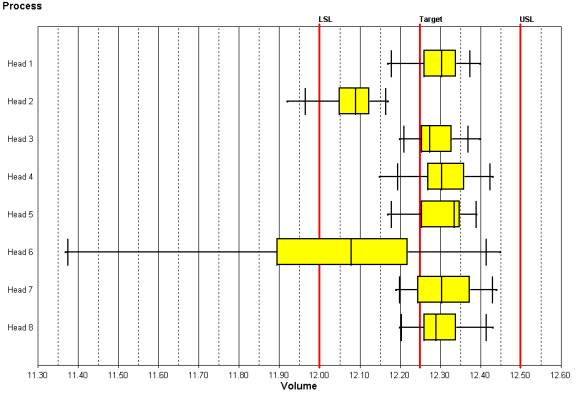Figure 4: Box Plot illustrating the fill volume distribution at each. Notice that heads 2 and 6 are filling to the low side with some falling below the lower limit.

Need help?
​For further information contact GetInTouch@infinityqs.com
Toll Free: 1.800.772.7978

### Take the first step from quality to excellence

Take the Next Steps